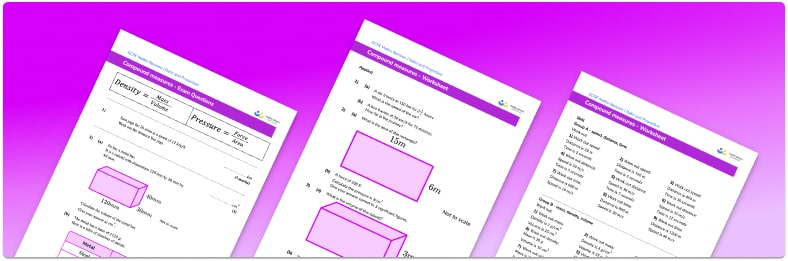# Compound Measures Worksheet• Section 1 of the compound measures worksheet  contains 27 skills-based compound measures questions, in 3 groups to support differentiation
• Section 2 contains 3 applied compound measures questions with a mix of worded problems and deeper problem solving questions
• Section 3 contains 4 foundation and higher level GCSE exam style compound measures questions
• Answers and a mark scheme for all compound measures questions are provided
• Questions follow variation theory with plenty of opportunities for students to work independently at their own level
• All questions created by fully qualified expert secondary maths teachers
• Suitable for GCSE maths revision for AQA, OCR, and Edexcel and WJEC exam boards

• This field is for validation purposes and should be left unchanged.

You can unsubscribe at any time (each email we send will contain an easy way to unsubscribe). To find out more about how we use your data, see our privacy policy.

### Compound measures at a glance

Compound measures are measures that use more than one unit.

Speed (Distance, Time)

Speed is found using the formula Speed = Distance divided by Time. The units for speed are therefore distance per unit of time. Examples include miles per hour or metres per second.

To find the average speed for a whole journey we would calculate the total distance divided by the total time.

Density (Mass, Volume)

Density is calculated using the formula Density – Mass divided by Volume. The units for density are therefore mass per unit of volume. Examples include g/cm3 and kg/m3, since the volume of cuboids, prisms and all 3D objects is measured in cubic units.

Pressure (Force, Area)

Pressure is calculated using the formula Pressure = Force divided by Area. The units are therefore force per unit of area. Examples include N/m2 and Pa (Pascals).

Other compound measures

Other measures that use compound units include rates of pay, for example £15/hour and rates of flow, for example 10 litres/min.

Looking forward, students can then progress to additional ratio and proportion worksheets, for example a ratio worksheet or a simplifying and equivalent ratios worksheet.

For more teaching and learning support on Ratio and Proportion our GCSE maths lessons provide step by step support for all GCSE maths concepts.

## Do you have KS4 students who need more focused attention to succeed at GCSE?There will be students in your class who require individual attention to help them succeed in their maths GCSEs. In a class of 30, it’s not always easy to provide.

Help your students feel confident with exam-style questions and the strategies they’ll need to answer them correctly with our dedicated GCSE maths revision programme.

Lessons are selected to provide support where each student needs it most, and specially-trained GCSE maths tutors adapt the pitch and pace of each lesson. This ensures a personalised revision programme that raises grades and boosts confidence.

Find out more